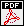# TU Lecture, WS 2007/08

## Random matrices and related problems

Content: In this lecture we will explore some aspects of random matrix theory. We will then see how the underlying mathematical structure can be applied to various models in mathematics and physics. These models span from directed percolation, random domino tiling, equilibrium and non-equilibrium statistical mechanics.

Program: October 15: It will be an introduction to the lecture and a rough plan of the content will be also presented.
October 22 - December 17: We introduce the relevant mathematical objects / methods using as a basis model one ensemble of random matrices.
January 7 - February 11: The rest of the semester will be devoted to a few applications. We will apply the mathematical structure we learned to a few models (non random matrix models).

Schedule:WS 2007/08, Monday 10-12, MA 645

Scanned lectures notes: (first versions, are not missprints free: take them with a grain of salt)

 • A single file of the lecture notes• References used in the preparation of the lecture notes• Introduction• Annexe to introduction: some pictures• The Classical Gaussian Ensembles of Random Matrices• Annexe: a variational formula• Gaussian Unitary Ensemble (GUE): determinantal correlation functions• Annexe: Cauchy-Binet and Christoffel-Darboux formulas• Universal scalings for GUE: Sine and Airy kernel• Annexe: Logarithmic fluctuations of sine kernel• Genaral point processes and determinantal point processes• Fredholm determinant, part 1• Annexe of functional analysis• Fredholm determinant, part 2• Tracy-Widom distribution and Painlevé II• Dnamics of point process: extended point processes• Non-intersecting Brownian bridges and the Airy process• Dyson's Brownian Motion and LGV theorem• Application to the 3D-Ising corner• Application to the PNG droplet, part 1• Application to the PNG droplet, part 2